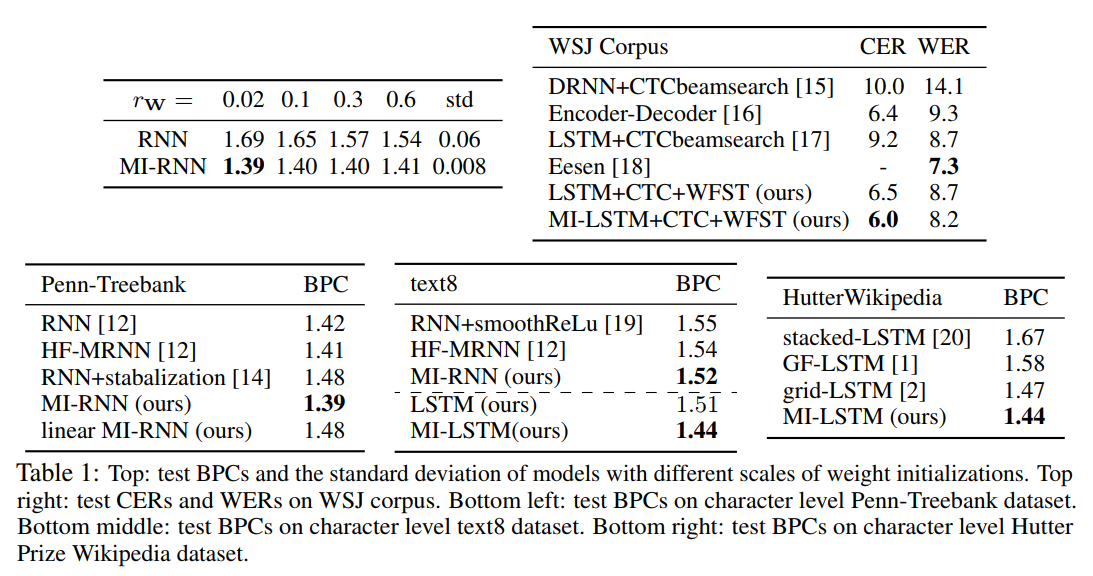## Main idea

Replace the RNN core additive block with a multiplicative block using the Hadamard product.

[Reminder] Hadamard product: $$(A \odot B)_{ij} = a_{ij} \times b_{ij}$$

Core block of Vanilla RNN vs Proposed Multiplicative-Integration RNN (MI-RNN):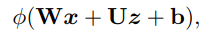$$\rightarrow$$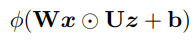In a more general form, where each matrix has its own bias term: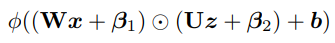Finally, with an added “gate” on the second order term: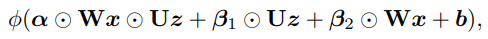(If $$\alpha = 0$$, we get back to the original additive block)

NOTES:

• Number of parameters is about the same
• Second-order term shares parameters with first-order terms
• Can be easily added to existing architectures (e.g. LSTM/GRU)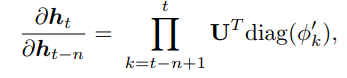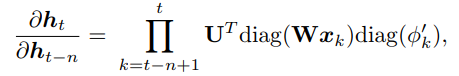(For the simple case. In the general case, we have: diag$$(\alpha \odot W x_k + \beta_1)$$)

NOTES:

• Gradient is now “gated” by $$\text{diag}(Wx_k)$$
• Gradient propagation is easier with $$Wx_k$$ involved

## Experiments using Penn-Treebank (text) dataset

### Activations problem

Activations over the validation set, using tanh as the nonlinearity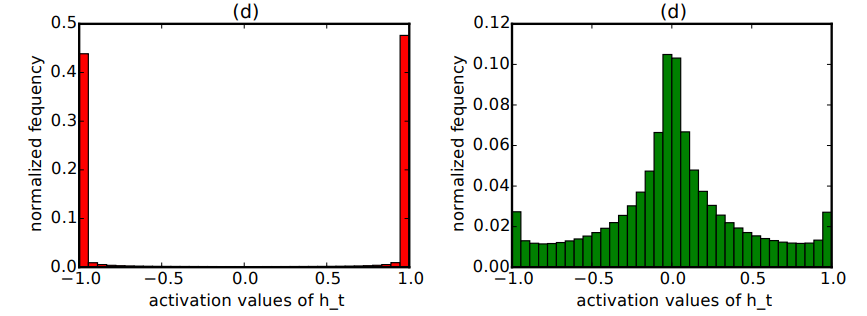[Reminder] Tanh derivative: $$\nabla \tanh(x) = 1 - \tanh^2(x)$$

• For saturated activations, $$\text{diag}(\phi'_k) \approx 0$$ (no gradient flow)
• For non-saturated, $$\text{diag}(\phi'_k) \approx 1$$

### Scaling problem

• Pre-activation term : $$Wx_k + Uh_{k-1}$$
• For one-hot vectors, $$Wx_k$$ is much smaller than $$Uh_{k-1}$$; initialization matters a lot! (See top-left of Fig.1, where $$r_w$$ is the uniform initialization range)

## Comparative experiments